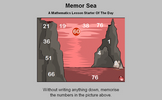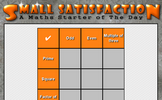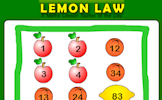# Multiplying and dividing fractions

### Term 1 starting in week 5 :: Estimated time: 2 weeks

• Represent multiplication of fractions
• Multiply a fraction by an integer
• Find the product of a pair of unit fractions
• Find the product of a pair of any fractions
• Divide an integer by a fraction
• Divide a fraction by a unit fraction
• Understand and use the reciprocal
• Divide any pair of fractions
• Multiply and divide improper and mixed fractions
• Multiply and divide algebraic fractions

This page should remember your ticks from one visit to the next for a period of time. It does this by using Local Storage so the information is saved only on the computer you are working on right now.

## Lesson Starters

Here are some suggestions for whole-class, projectable resources which can be used at the beginnings of each lesson in this block.

### 1st Lesson#### Memor Sea

Twelve numbers in a seascape need to be memorised in order to answer the five questions.

### 2nd Lesson#### Small Satisfaction

Arrange the digits one to nine in the grid so that they obey the row and column headings.

### 3rd Lesson#### Lemon Law

Change the numbers on the apples so that the number on the lemon is the given total.

### 4th Lesson#### Letters in a Number

Questions about the number of letters in numbers.

### 5th Lesson#### Consecy Brothers

Which three consecutive numbers multiplied together give the given answer.

### 6th Lesson#### Eleven Plus Two

Rearrange the letters in the expression 'Eleven Plus Two' to make another expression with the same value.

Some of the Starters above are to reinforce concepts learnt, others are to introduce new ideas while others are on unrelated topics designed for retrieval practice or and opportunity to develop problem-solving skills.

White Rose ResourcesEnd of block assessments provide a quick progress check at the end of each block of learning to make sure students have understood the content covered. This Scheme of Learning was produced by White Rose Maths and is used here with permission granted on 30th June 2021.For All: